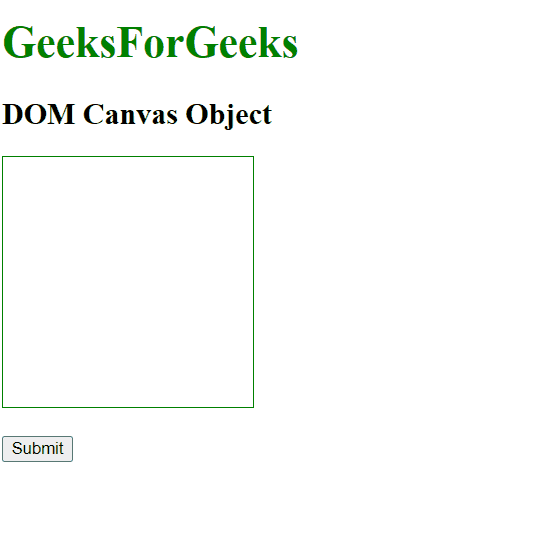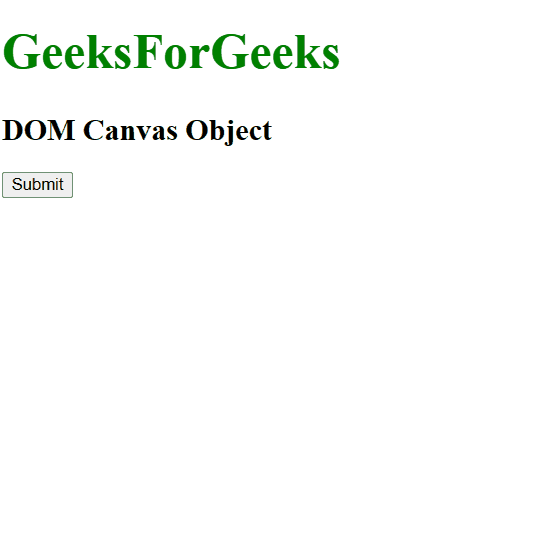# HTML DOM Canvas Object

The DOM Canvas Object is used to represent the HTML <Canvas> element. The <canvas> tag is used to draw graphics in the document using javascript. It is new in HTML5. The canvas element is accessed by getElementById()

Syntax:

`accessed by getElementById("id"). `

Where “id” is the ID assigned to the Canvas tag.

Example 1: In this example, we will use the DOM Canvas Object.

## HTML

 `` `<``html``>` `<``head``>` `    ``<``title``>canvas Tag` `` `  `  `<``body``>` `        ``<``h1` `style``=``"color:green; Font-size:35px;"``>` `            ``GeeksForGeeks` `        ``` `        ``<``h2``>DOM Canvas Object`   `        ``` `        ``<``canvas` `id``=``"geeks"` `                ``height``=``"200"` `                ``width``=``"200"` `                ``style``=``"border:1px solid green"``>` `        ``` `  `  `        ``` `        ``<``br``>` `        ``<``br``>` `        ``<``button` `onclick``=``"myGeeks()"``>Submit` `        ``<``script``>` `            ``function myGeeks() {` `            ``let gfg = document.getElementById("geeks");` `            ``let sudo = gfg.getContext("2d");` `                ``sudo.beginPath();` `                ``sudo.arc(100, 100, 90, 0, 2 * Math.PI);` `                ``sudo.stroke();` `            ``}` `        ``` `` ``

Output:Example 2: Canvas Object can be created by using the document.createElement method.

## HTML

 `` `<``html``>` `<``head``>` `    ``<``style``>` `        ``canvas {` `            ``border: 3px solid black;` `        ``}` `    ``` `` `  `  `<``body``>` `    ``<``h1` `style``=``"color: green; font-size:40px;"``>` `        ``GeeksForGeeks` `    ``` `    ``<``h2``>DOM Canvas Object` `    ``<``button` `onclick``=``"myGeeks()"``>` `        ``Submit` `    ``` `    ``<``script``>` `        ``function myGeeks() {` `            ``let geeks = document.createElement("CANVAS");` `            ``let gfg = geeks.getContext("2d");` `            ``gfg.fillStyle = "green";` `            ``gfg.fillRect(40, 40, 200, 100);` `            ``document.body.appendChild(geeks);` `        ``}` `    ``` `` ``

Output:Supported Browsers: The browser supported by DOM Canvas Object are listed below: